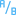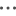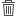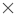# Calculate a field

You can add new fields to your dataset using the View Data Table window. View Data Table allows you to add new fields, such as growth rates, percent loss, and change over time, by choosing fields from your dataset and applying operators and functions.

##### Note:
• The data table provides a representative view of your data, and has a display limit of 2,000 rows. Sorting your table in ascending and descending order allows you to view the top 2,000 rows, and the bottom 2,000 rows. All calculations will be performed on the full dataset.
• The new calculated field only appears in your workbook, not in the original dataset. For example, after adding a calculated field of percentchange to a CommodityPrices dataset added from Microsoft Excel, the percentchange field is available in your workbook but is not added to the original Excel file. If you want to preserve the calculated field outside of the workbook, you can create a new feature layer by sharing the dataset.
• You can also calculate fields using Calculate % Change, Calculate Ratio, and Calculate Z-Score in Find answers.
##### Tip:

Use View Data Table to add normalized data to your dataset for maps, charts, and tables. After the data is calculated and added your dataset, change the field role to identify it as a rate/ratio field.

## Add a field to your dataset

1. From the data pane, click the Dataset options buttonnext to the dataset where you want to add a calculated field.
2. Click View Data Table.
3. Click + Field.

A column called New Field is appended to the table.

##### Note:

You can resize and reorder the columns, but these changes are not saved.

4. Click the heading of the new column and provide a more descriptive name.
5. Click fx or Enter calculate function to display a menu with functions, field names, and operators to build your formula. Equivalent keyboard commands can also be used to replace any of the buttons from the fx menu.
6. Use the functions, fields, and operators to complete your calculation as required.
7. Click Run. If the Run button is not enabled, then there is an error in the syntax of your calculation.
8. The new calculated field appears at the bottom of your dataset. You can remove a calculated field by selecting it and clicking the Delete calculated field button.

## Operators

Calculating a field can require both mathematical and logical operators. The following operators are available for calculating fields:

OperatorsUse

+

-

Subtraction

×

Multiplication. The equivalent keyboard command is *.

÷

Division. The equivalent keyboard command is /.

xy

Power function. The equivalent keyboard command is ^.

<

Less than

>

Greater than

=

Equal to

<=

Less than or equal to

>=

Greater than or equal to

<>

Not equal to

,

Comma, used as a separator between syntax components in functions.

(

Left bracket

)

Right bracket

AND

Logical operator where all conditions must be met.

OR

Logical operator where one of the conditions must be met.

The AND and OR operators can be used to join conditions with different syntax than their corresponding logical functions. The following examples show equivalent calculations using the functions and operators:

##### Note:

When calculating fields, AND and OR must be used within the IF() function.

FunctionOperator

IF(AND(MeanAge>=18, MeanAge<=33),"Millennial","Other")

IF(MeanAge>=18 AND MeanAge<=33,"Millennial","Other")

IF(OR(Crime="Theft", Crime="Theft from vehicle", Crime="Shoplifting"), "Larceny", "Other")

IF(Crime="Theft" OR Crime="Theft from vehicle" OR Crime="Shoplifting", "Larceny", "Other")

## Functions

Functions can be accessed using the fx button or the Enter calculate function field in the Data Table. There are four types of functions: string, numeric, date, and logical.

When you add a function to your calculation, a pop-up will be displayed with the function syntax. You can remove the pop-up using the close dialog button, or redisplay the pop-up by clicking on the function in the Enter calculate function field.

### String functions

Most string functions use string inputs to produce string outputs. The two exceptions are the VALUE() function and the FIND() function, which both use string inputs to produce numeric outputs.

Input text in string functions can be either literal (text surrounded by quotation marks) or category field values. The following table uses category fields for its examples, along with example values that could be found in those fields. Quotation marks are used in the field values to demonstrate that the fields have categorical data.

SyntaxDescriptionExample

CONCATENATE(text1, [text2], ... )

Concatenates two or more string values.

A dataset of schools in California contains fields for street address, city, and ZIP Code. A single field of mailing addresses can be created using the CONCATENATE() function:

• Function syntax: CONCATENATE(Address,", ", City," , CA, ",ZIP)
• Example field values:
• Address = "380 New York St"
• City = "Redlands"
• ZIP = "92373"
• Result text: "380 New York St, Redlands, CA, 92373"

FIND(find_text, within_text, [start_num])

Gives the position of the specified text (character or characters) within a string or text field. The FIND() function may be most useful when used in conjunction with other functions, such as MID(), LEFT(), or RIGHT().

A dataset contains a field with street addresses (including number and street name). In order to classify the data by street, the street name has to be removed from the rest of the address using the MID() function. However, each number is a different length, so the start_num will be different for each row. The start_num can be found using the FIND() function:

• Function syntax: FIND(" ", Address)
• Example field values:
• Address = "380 New York St"
• Result number: 4

LEFT(text, [num_chars])

Returns part of a text field.

• num_chars: specifies how many characters of the expression will be returned and should be an integer. The number of characters will be counted from left to right, starting in the first position. If num_chars is not provided, the default will be one character.

A dataset of traffic accidents includes a category field with the day of the accident, including the day of the week, date, and year. In order to study the accidents by the day of the week, a new field can be calculated to show the first three characters of the field (starting with day of the week) using the LEFT() function:

• Function syntax: LEFT(Accident_Date, 3)
• Example field values:
• Accident_Date = "Monday, November 14, 2016"
• Result text: "Mon"

LOWER(text)

Returns a character expression with all data converted to lowercase.

A public works department is compiling a list of street signs that need to be replaced. As new entries are added to the list, the format of the Status field has become unstandardized, making it difficult to display the signs with unique values. The Status field can be standardized to have all lowercase letters using the LOWER() function:

• Function syntax: LOWER(Status)
• Example field values:
• Status = "Installed"
• Result text: "installed"

MID(text, start_num, [num_chars])

Returns part of a text field.

• start_num: specifies the position of the first character (starting at 1). The start_num must be an integer.
• num_chars: specifies how many characters of the expression will be returned and should be an integer. No empty characters are returned if the num_chars value is greater than the length of the string. If num_chars is not provided, the default will be all the characters after the starting character.

A dataset of schools in California contains fields for street address, city, and ZIP Code. The street name can be isolated from the street address using the MID() function:

• Function syntax: MID(Address, 5, 20)
• Example field values:
• Address = "380 New York St"
• Result text: "New York St"

RIGHT(text, [num_chars])

Returns part of a text field.

• num_chars: specifies how many characters of the expression will be returned and should be an integer. The number of characters will be counted from right to left, starting in the last position. If num_chars is not provided, the default will be one character.

A dataset of national parks includes a field with the name of the park and the two digit state code. To symbolize the parks by state, a new field can be added and calculated using the RIGHT() function:

• Function syntax: RIGHT(Park, 2)
• Example field values:
• Park = "Hawai'i Volcanoes National Park, HI"
• Result text: "HI"

SUBSTITUTE(source_text, old_text, new_text)

Replaces the old text in a string with the specified new text.

• source_text: The original text or field with text.
• old_text: The text within the source_text that you want to replace.
• new_text: The text to replace the old_text.

A dataset of pothole locations contains a field with street names. The dataset needs to be updated when Main Street is renamed 5th Street. The street_name field can be updated using the SUBSTITUTE() function:

• Function syntax: SUBSTITUTE(street_name, "Main", "5th")

##### Note:

The SUBSTITUTE() function will replace all occurrences of old_text with new_text. For example, in the function SUBSTITUTE("aba", "a", "c") the result text would be "cbc".

TRIM(text)

Returns the string with extra spaces removed from the ends.

A feature service contains text fields with extra spaces at the beginning and end of their values. The extra spaces can be removed using the TRIM() function:

• Function syntax: TRIM(City)
• Example field values:
• City = " Redlands "
• Result text: "Redlands"

UPPER(text)

Returns a character expression with all data converted to uppercase.

A dataset with locations of NGO headquarters contains a field with the organizations' full names and their acronyms when applicable. The acronyms can be standardized to have all capital letters using the UPPER() function:

• Function syntax: UPPER(Org)
• Example field values:
• Org = "Spew"
• Result text: "SPEW"

VALUE(text, [format])

Converts text to a number.

• format: specifies the character used as a decimal separator in the number. The format may be either a comma (",") or dot ("."). If a format is not provided, the default separator will be a dot.

##### Note:

Nested functions within the VALUE() function may output unexpected results if a format is not provided. Therefore, it is recommended that you specify a format when using the VALUE() function.

The VALUE() function does not currently support converting text to negative numbers.

A dataset of retail stores has a category field with the revenue amounts. The Revenue field can be converted to numerical values using the VALUE() function.

• Function syntax: VALUE(Revenue, ".")
• Example field values:
• Revenue = "1,000.00"
• Result number: 1000.00

### Numeric functions

Numeric functions use number inputs to produce number outputs. Number functions are most likely to be used in conjunction with other functions or as a method of transforming data.

Input numbers can be either literal numbers or number fields. Some of the examples below use numbers as input rather than fields to better display the usage of each function.

SyntaxDescriptionExample

ABS(number)

Returns the absolute value.

• Function syntax: ABS(-350)
• Result number: 350

AVG(number)

Returns the mean value.

• Function syntax: AVG(field)
• Example number values for field: 4, 5, 11, 6.5
• Result: 6.63

CEILING(number)

Rounds a number up to the nearest multiple of 1.

• Function syntax: CEILING(7.8)
• Result number: 8

COS(number)

The trigonometric cosine of the specified angle, in radians.

The following formula can be used to convert degrees to radians:

``````radians = θπ/180
where:
θ = the angle in degrees
π ≈ 3.14``````

• Function syntax: COS(0.35)
• Result number: 0.94

FLOOR(number)

Rounds a number down to the nearest multiple of 1.

• Function syntax: FLOOR(7.8)
• Result number: 7

LN(number)

The natural logarithm of the specified float expression. The natural logarithm uses the constant e as the base value (approximately 2.72)

• Function syntax: LN(16)
• Result number: 2.77

LOG(number)

The logarithm of a number to a specific base. The default is base 10.

• Function syntax: LOG(16, 2)
• Result number: 4

MAX(number)

Returns the maximum value.

• Function syntax: MAX(field)
• Example number values for field: 4, 5, 11, 6.5
• Result: 11

MIN(number)

Returns the minimum value.

• Function syntax: MIN(field)
• Example number values for field: 4, 5, 11, 6.5
• Result: 4

POWER(number, power)

The value of the expression raised to the specified power.

• Function syntax: POWER(2, 4)
• Result number: 16

ROUND(number, num_digits)

Rounds numeric values to the specified number of digits.

• num_digits = the number of decimal places in the output
• If num_digits is positive, the number is rounded to the corresponding number of decimal places
• If num_digits is zero, the number is rounded to the nearest integer
• If num_digits is negative, the number is rounded to the specified number of digits left of the decimal point

• Function syntax: ROUND(54.854827, 2)
• Result number: 54.85
• Function syntax: ROUND(54.854827, -1)
• Result number: 50

SIN(number)

The trigonometric sine of the specified angle, in radians.

The following formula can be used to convert degrees to radians:

``````radians = θπ/180
where:
θ = the angle in degrees
π ≈ 3.14``````

• Function syntax: SIN(0.79)
• Result number: 0.71

SUM(number)

Returns the total value.

• Function syntax: SUM(field)
• Example number values for field: 4, 5, 11, 6.5
• Result: 26.5

TAN(number)

Returns the tangent of the input expression.

The following formula can be used to convert degrees to radians:

``````radians = θπ/180
where:
θ = the angle in degrees
π ≈ 3.14``````

• Function syntax: TAN(1.05)
• Result number: 1.74

### Date functions

Date functions can use date fields, text, or no input, depending on which function is being used. The DATEVALUE() function can be used to replace a date field in the DATEDIF() function.

SyntaxDescriptionExample

DATE(year, month, day, [hour, minute, second], [AM/PM])

Creates a date/time field using three or more separate fields or values. The following specifications are accepted:

• year: "YY" (two digit year) or "YYYY" (four digit year)
• month: "MM" (1-12)
• day: "DD" (1-31)
• hour: "HH" (0-23) or "hh" (1-12)
• minute: "mm" (0-59)
• second: "ss" (0-59)
• AM/PM: "AM"/"PM" (not case sensitive)

##### Note:

All input fields can be numbers or strings, except the AM/PM parameter. Numbers must be integer values.

If you are using the DATE() function with a database dataset and you have specified any time parameters (hour, minute, or second), you must enter an input value for all time parameters.

A dataset of restaurants includes string fields with the date and time of the most recent health inspections. A date field for the inspections can be created using the DATE() function:

• Function syntax: DATE(2016, Month, Day, Hour, Min, 00)
• Example field values:
• Month = 8
• Day = 15
• Hour = 11
• Minute = 30
• Result date: 8/15/2016, 11:30:00

##### Note:

The result dates in this example will be formatted using a 24-hour clock. To use a 12-hour clock, you must specify AM or PM. The same example function could be written as DATE(2016, Month, Day, Hour, Min, 00, "AM")

DATEDIF(start_date, end_date, unit)

Calculates the elapsed time between two dates. The start_date must take place before the end_date, otherwise the output will be a negative value.

• start_date and end_date can be date fields or a DATEVALUE() function.
• unit: the unit of time to be returned. The supported unit values include:
• "ss" = seconds (default)
• "mm" = minutes
• "h" = hours
• "D" = days
• "M" = months
• "Y" = years

A dataset of restaurants includes the dates of their most recent health inspections. The number of days elapsed between health inspections can be calculated using the DATEDIF() function:

• Function syntax: DATEDIF(Inspection1, Inspection2, "D")
• Example field values:
• Inspection1 = 8/15/2016, 11:30:00 AM
• Inspection2 = 10/31/2016 2:30:00 PM
• Result number: 77

DATEVALUE(date_text, [format])

Converts text to a date.

• date_text can be a string field or text entered inside quotation marks.
• format (optional) - The format of the date entered. The format is entered as text surrounded by quotation marks. The format can be entered using the following unit specifications:
• "MM" - Month (1-12)
• "DD" - Day of the month (1-31)
• "YY" - Two digit year
• "YYYY" - Four digit year
• "HH" - Hour (0-23)
• "hh" - Hour (1-12)
• "mm" - Minutes (0-59)
• "ss" - Seconds (0-59)
• "AM"/"PM" - Not case sensitive

##### Note:

Nested functions within the DATEVALUE() function may output unexpected results if a format is not provided. Therefore, it is recommended that you specify a format when nesting functions within DATEVALUE().

Dates specified without separators (for example, "10312016") will be treated as milliseconds if no format is provided. Therefore, it is recommended that you specify a format when entering dates without separators.

For dates specified with separators but without a format, the format will be guessed. If the format cannot be guessed, the default "MM-DD-YY" will be applied.

Except for "AM"/"PM", letters cannot be used to specify dates. Therefore, dates such as "31 Oct 2016" will not be supported.

The DATEVALUE() function can only read text entered inside quotation marks or string fields. A date/time field cannot be used as input to the DATEVALUE() function.

A dataset of restaurants includes the dates of their most recent health inspections. The number of days elapsed since the last inspection can be calculated using the DATEVALUE() function to specify the current date:

• Function syntax: DATEDIF(Inspection_date, DATEVALUE("10/31/2016", "DD/MM/YYYY"), "D")
• Example field values:
• Inspection_date = 8/15/2016, 11:30:00 AM
• Result number: 77

Dates and times can be formatted in several ways. The following examples are some of the ways you can format text into dates:

• DATEVALUE("12/25/2016 12:30:25 pm", "MM/DD/YYYY hh:mm:ss pm")
• DATEVALUE("12/25/2016 14:23:45 pm", "MM/DD/YYYY HH:mm:ss AM")
• DATEVALUE("25-08-2008 08:40:13 am", "DD/MM/YYYY hh:mm:ss PM")

NOW()

Returns the current date and time in date/time format. The time is recorded based on Coordinated Universal Time (UTC).

A dataset of restaurants includes the dates of their most recent health inspections. The number of days elapsed since the last inspection can be calculated using the NOW() function to specify the current date:

• Function syntax: DATEDIF(Inspection_date, NOW(), "D")
• Example field values:
• Inspection_date= 8/15/2016, 11:30:00 AM
• Result number: 77

### Logical functions

Logical functions use string or number inputs to produce string or number outputs. The inputs can be either literal (text surrounded by quotation marks or constant numbers) or field values. The following table uses a combination of field and literal values for its examples, along with example values that could be found in the given fields.

SyntaxDescriptionExample

AND(condition,condition, ...)

Tests two or more conditions and returns TRUE if all conditions are met. AND() should be used within the condition parameter of the IF() function.

• condition: any expression that includes at least one conditional operator. The condition can include numeric, string, and field values. The following conditional operators can be used:
• >: greater than
• <: less than
• >=: greater than or equal to
• <=: less than or equal to
• =: equal to
• <>: not equal to

A dataset contains the mean age of people living within each block group. A market researcher wants to know which block groups contain mostly millennials. The block groups with a mean age within the age range for millennials can be found using a combination of the IF() and AND() functions:

• Function syntax: IF(AND(MeanAge>=18, MeanAge<=33),"Millennial","Other")
• Example field value:
• MeanAge = 43
• Result text: "Other"

IF(condition, TRUE_expression, [FALSE_expression])

Tests a condition and returns a TRUE or FALSE value based on the result.

• condition: any expression that includes at least one conditional operator. The condition can include numeric, string, and field values. The following conditional operators can be used:
• >: greater than
• <: less than
• >=: greater than or equal to
• <=: less than or equal to
• =: equal to
• <>: not equal to
• TRUE_expression: the expression that will be used if the condition is true. This parameter is mandatory.
• FALSE_expression: the expression that will be used if the condition is false. If no FALSE_expression is provided, the default will be null.

TRUE_expression and FALSE_expression can be any valid expression, including a nested IF() function. The data type for TRUE_expression and FALSE_expression should be the same type (for example, both strings or both numbers).

A dataset contains fields with store revenue and expenses, which can be used to find the status of the stores (whether they have posted a net profit or a net loss). The status of each store can be found using the IF() function:

• Function syntax: IF(Revenue>Expenses, "Profit","Loss")
• Example field values:
• Revenue = 400,000
• Expenses = 350,000
• Result text: "Profit"

OR(condition,condition, ...)

Tests two or more conditions and returns TRUE if at least one condition is met. OR() should be used within the condition parameter of the IF() function.

• condition: any expression that includes at least one conditional operator. The condition can include numeric, string, and field values. The following conditional operators can be used:
• >: greater than
• <: less than
• >=: greater than or equal to
• <=: less than or equal to
• =: equal to
• <>: not equal to

A dataset contains locations of crimes across a city. A crime analyst wants to analyze the pattern of larceny compared to other similar crimes. To compare larceny to other crimes, the analyst must first determine whether or not each crime was considered larceny. The type of crime can be determined using a combination of the IF() and OR() functions:

• Function syntax: IF(OR(Crime="Theft", Crime="Theft from vehicle", Crime="Shoplifting"), "Larceny", "Other")
• Result text: "Larceny"